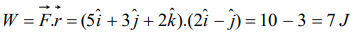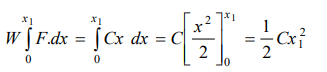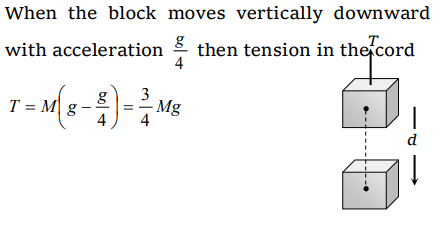## Work, Energy and Power Questions and Answers Part-4

1. A ball of mass m moves with speed v and strikes a wall having infinite mass and it returns with same speed then the work done by the ball on the wall is
a) Zero
b) $mv$ J
c) $m\diagup v.J$
d) $v\diagup m$ J

Explanation: The ball rebounds with the same speed. So change in it's Kinetic energy will be zero i.e. work done by the ball on the wall is zero

2.A force $\vec{F}=\left(5\hat{i}+3\hat{j}+2\hat{k}\right)$   N is applied over a particle which displaces it from its origin to the point $\vec{r}=\left(2\hat{i}-\hat{j}\right)$   m . The work done on the particle in joules is
a) – 7
b) +7
c) +10
d) +13

Explanation:3. The kinetic energy acquired by a body of mass m is travelling some distance s, starting from rest under the actions of a constant force, is directly proportional to
a) $m^{0}$
b) m
c) $m^{2}$
d) $\sqrt{m}$

Explanation:4.If a force $\vec{F}=4\hat{i}+5\hat{j}$   causes a displacement $\vec{s}=3\hat{i}+6\hat{k}$   , work done is
a) $4 \times6$  unit
b) $6 \times3$   unit
c) $5 \times6$   unit
d) $4 \times3$  unit

Explanation:5. A man starts walking from a point on the surface of earth (assumed smooth) and reaches diagonally opposite point. What is the work done by him
a) Zero
b) Positive
c) Negative
d) Nothing can be said

Explanation: As surface is smooth so work done against friction is zero. Also the displacement and force of gravity are perpendicular so work done against gravity is zero.

6. It is easier to draw up a wooden block along an inclined plane than to haul it vertically, principally because
a) The friction is reduced
b) The mass becomes smaller
c) Only a part of the weight has to be overcome
d) ‘g’ becomes smaller

Explanation: Opposing force in vertical pulling = mg
But opposing force on an inclined plane is mg sin $\theta$, which is less than mg.

7. Due to a force of $\left(6\hat{i}+2\hat{j}\right)$   N the displacement of a body is $\left(3\hat{i}-\hat{j}\right)m$  , then the work done is
a) 16 J
b) 12 J
c) 8 J
d) Zero

Explanation:8. A ball is released from the top of a tower. The ratio of work done by force of gravity in first, second and third second of the motion of the ball is
a) 1 : 2 : 3
b) 1 : 4 : 9
c) 1 : 3 : 5
d) 1 : 5 : 3

Explanation:9. A particle moves under the effect of a force F = Cx from $x=0$ to $x=x_{1}$ . The work done in the process is
a) $Cx_1^2$
b) $\frac{1}{2}Cx_1^2$
c) $Cx_1$
d) Zero10. A cord is used to lower vertically a block of mass M by a distance d with constant downward acceleration$\frac{g}{4}$. Work done by the cord on the block is
a) $Mg\frac{d}{4}$
b) $3Mg\frac{d}{4}$
c) $-3Mg\frac{d}{4}$